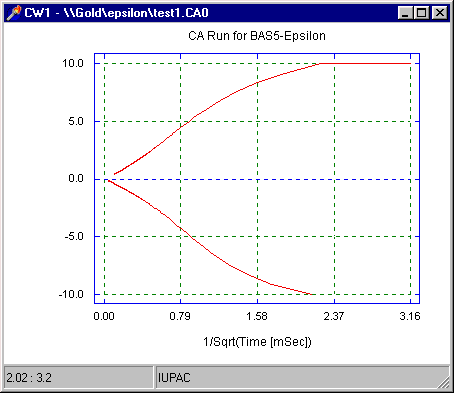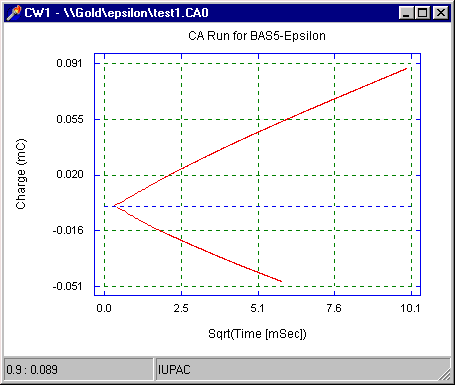# Chronoamperometry/chronocoulometry - Data Analysis

The analysis of chronoamperometry (CA) data is based on the Cottrell equation, which defines the current-time dependence for linear diffusion control:

i = nFACD½pt

 where: n = number of electrons transferred/molecule F = Faraday's constant (96,500 C mol-1) A = electrode area (cm2) D = diffusion coefficient (cm2 s-1) C = concentration (mol cm-3)

This indicates that, under these conditions, there is a linear relationship between the current and the 1/square root of time. A plot of i vs. t is often referred to as the Cottrell plot. This plot is available in the epsilon software by selecting I vs 1/sqrt(T) from Select Graph in the pop-menu.

The analysis of chronocoulometry (CC) data is based on the Anson equation, which defines the charge-time dependence for linear diffusion control:

Q = 2nFACD½pt ½

Therefore, under these conditions, there is a linear relationship between the charge and the square root time. A plot of Q vs. t½ is often referred as the Anson plot. This plot is available in the epsilon software by selecting Q vs sqrt(T) from Select Graph in the pop-menu.

The slope and intercept of the Cottrell and Anson plots can be measured by the epsilon software (click here for details). Since the slope of the Cottrell and Anson plots are determined by n, A, D, and C, one of these parameters can be calculated from the slope, provided the other three are known. One application of CA and CC is the determination of either D or A.

It is important to note that in any potential step experiment, there is a delay in attaining the step potential due to the finite rise time of the potentiostat. This non-ideal behavior affects the validity of the data points measured during this delay time, and hence these data are discarded when calculating the slope and intercept (the default condition for the epsilon software is that the final 80 % of data points are used). The non-linearity of the early data points is most clearly shown in the Cottrell plot (Fig1), due to the reciprocal time function (i.e., early data points = large x). In contrast, the non-linearity is much less pronounced in the Anson plot (Fig2).Figure 1. Cottrell plot for a chronoamperometry experiment.Figure 2. Anson plot for a chronocoulometry experiment.

Double potential step techniques can be used to investigate the kinetics of chemical reactions following electron transfer. As discussed elsewhere, only a fraction of the molecules of O that are formed as a result of the first potential step are reduced again during the second step. Therefore, the current or charge due to the second step (ir or Qr) is less than that due to the first step (if or Qf). If O undergoes a chemical reaction to a molecule that is not reduced after the second step, then even fewer molecules of O are available for reduction, and ir and Qr show a corresponding decrease. The rate of the chemical reaction (k) can be calculated by investigating the effect of changing the Step Time on the ir/if (or Qr/Qf) ratio and comparing these values to published working curves. Although working curves are available for both ir/if and Qr/Qf, CC is generally more favored due to the better signal to noise ratio.

One of the major applications of CC is the study of species adsorbed to the surface of the working electrode (indeed, it was originally devised for such studies). The advantage of using CC rather than CA is that it is possible to separate the charge due to the electrolysis of the adsorbed molecules from the charge due to the electrolysis of molecules in solution and the double layer charge (the analogous separation of currents is generally not possible). This is achieved using the Anson plot.

As discussed above, the electrolysis of solution species is diffusion-controlled, and depends on t½. In contrast, the electrolysis of adsorbed species is essentially instantaneously, as is the double layer charging. The equation for the total charge Q is:

Q = Qdiff + Qads + Qdl

or

Q = 2nFACD½pt½ + nFAG0 + Qdl

 where: Qdiff = charge due to electrolysis of solution species Qads = charge due to electrolysis of adsorbed species Qdl = double-layer charging G0 = surface concentration of adsorbed species (mol cm-2)

Therefore, the intercept of the Anson plot is the sum of Qdl and Qads. One method for eliminating Qdl from the equation is to run the identical experiment on the electrolyte alone. However, this approach assumes that Qdl is the same both in the presence and in the absence of the adsorbed analyte, which is generally not a valid assumption. The alternative method is to use the double potential step experiment. If only one of O and R adsorbs, then Qads is the difference of the intercepts of the Anson plots for the two steps.

It is important to note that data from the later time domains of the experiment are being used to investigate behavior that occurred at early time points. This shows that integration retains information about electrolysis that occurs essentially simultaneously with the potential step. This is a major advantage of CC, since direct measurement of such behavior is generally very difficult.

In all the above applications, the initial potential is at a value at which electrolysis does not occur, and the step potential is at a value at which electrolysis occurs at a diffusion-controlled rate (the second step is generally from the step potential back to the initial potential). Therefore, before these potentials can be determined, the redox potential must first be known. In general, the simplest way to find these potentials is to record the cyclic voltammogram of the analyte.